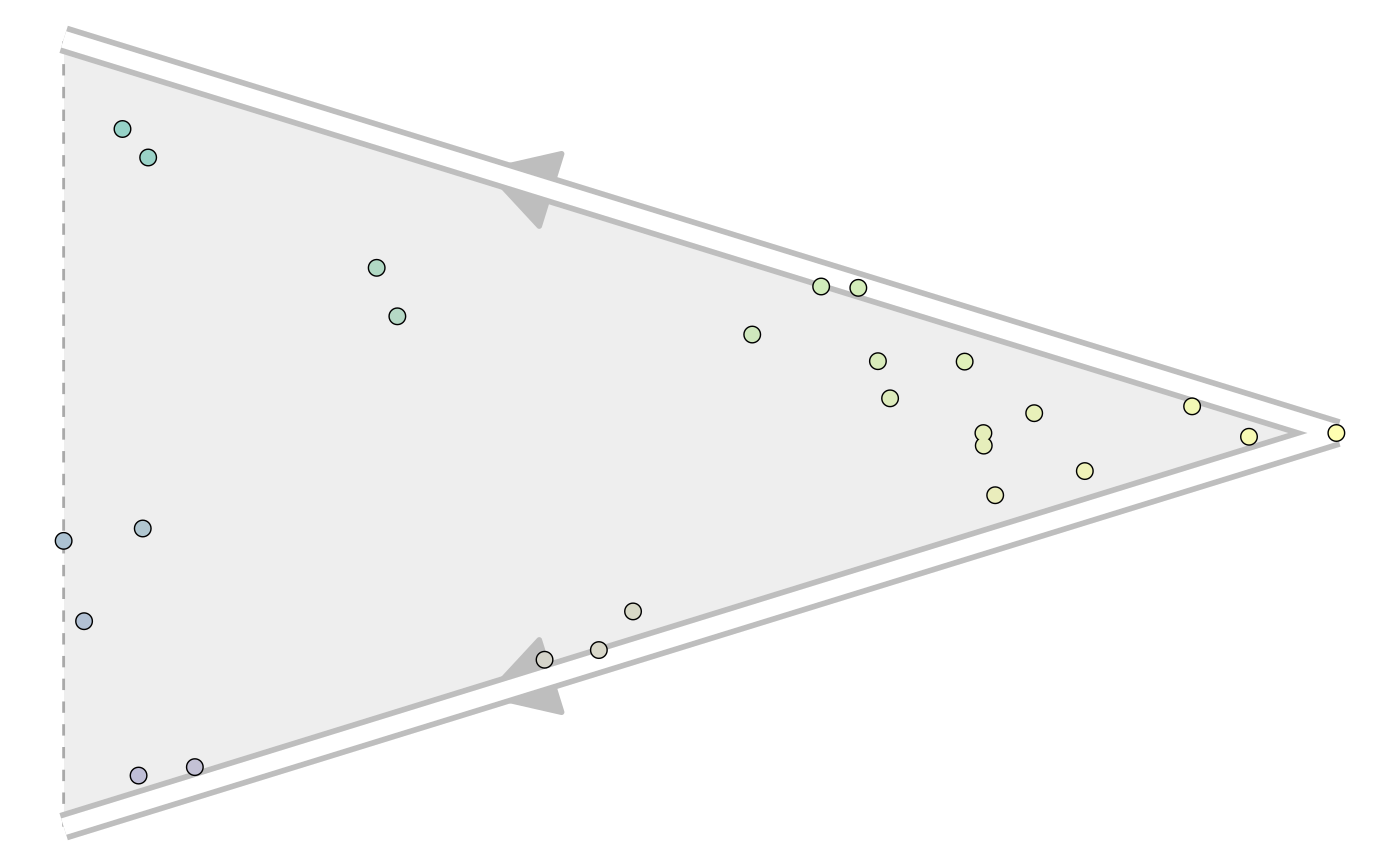# Multifurcating trajectory with end state probabilities

Constructs a multifurcating trajectory using the pseudotime values of each cell and their end state probabilities. If pseudotime values are not given, will use pseudotime already present in the dataset.

```add_end_state_probabilities(dataset, end_state_probabilities,
pseudotime = NULL, do_scale_minmax = TRUE, ...)```

## Arguments

dataset A dataset created by `wrap_data()` or `wrap_expression()` A dataframe containing cell_id (character) and additional numeric columns containing the probability for every end milestone. If the data_frame contains only a cell_id column, the data will be processed using `add_linear_trajectory` A named vector of pseudo times. Whether or not to scale the pseudotime between 0 and 1. Otherwise, will assume the values are already within that range. Extras to be added to the trajectory

The trajectory

## Details

This function will generate the milestone_network and progressions.

## Examples

```library(tibble)
dataset <- wrap_data(cell_ids = letters)

pseudotime <- runif(length(dataset\$cell_ids))
names(pseudotime) <- dataset\$cell_ids
pseudotime#>          a          b          c          d          e          f          g          h          i          j          k
#> 0.26208100 0.31291465 0.90985206 0.29921090 0.37633529 0.10503234 0.29894843 0.58034821 0.91686555 0.87572983 0.36744064
#>          l          m          n          o          p          q          r          s          t          u          v
#> 0.29059605 0.41783442 0.91381484 0.55536681 0.39066318 0.22506533 0.46822548 0.95670465 0.14662831 0.72767226 0.92862377
#>          w          x          y          z
#> 0.62005017 0.04116467 0.74278406 0.97165338 end_state_probabilities <- tibble(
cell_id = dataset\$cell_ids,
A = runif(length(dataset\$cell_ids)),
B = 1-A
)
end_state_probabilities#> # A tibble: 26 x 3
#>    cell_id      A     B
#>    <chr>    <dbl> <dbl>
#>  1 a       0.606  0.394
#>  2 b       0.810  0.190
#>  3 c       0.875  0.125
#>  4 d       0.500  0.500
#>  5 e       0.753  0.247
#>  6 f       0.430  0.570
#>  7 g       0.442  0.558
#>  8 h       0.0242 0.976
#>  9 i       0.0376 0.962
#> 10 j       0.0268 0.973
#> # … with 16 more rowstrajectory <- add_end_state_probabilities(dataset, end_state_probabilities, pseudotime)

# for plotting the result, install dynplot
dynplot::plot_graph(trajectory)#> Coloring by milestone#> Using milestone_percentages from trajectory```